# Angle of twist and Shear stress in a shaft

• Engineering
• Ben_Walker1978
In summary, the numerical values are correct this time, however, the maximum shear stress near the surface of the shaft should be 0.229 MPa (millions of Pascals) rather than GPa (billions of Pascals).

#### Ben_Walker1978

Homework Statement
Find the Angle of twist and Shear stress in a shaft
Relevant Equations
Angle of twist and Shear stress in a shaft
Hello,

I have attempted the question below.

I think i have correctly completed the question.

Could anyone see if i have gone wrong?

As trying to learn this.

I am just worried about the units m to mm. Also the answer being correct, 917.6Gpa. I was unsure what units to use on the answer.

Question: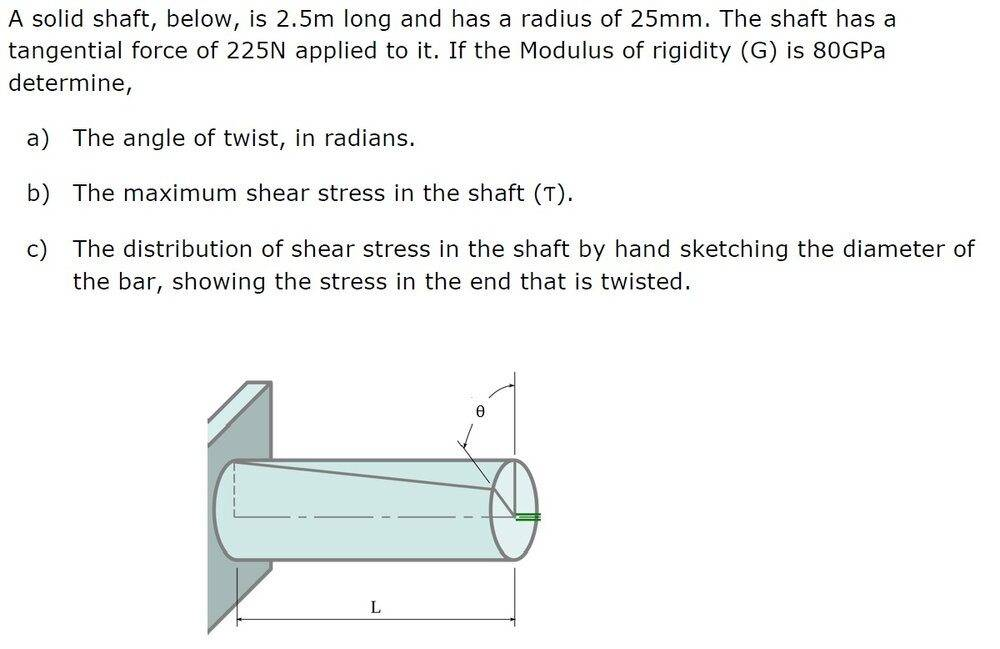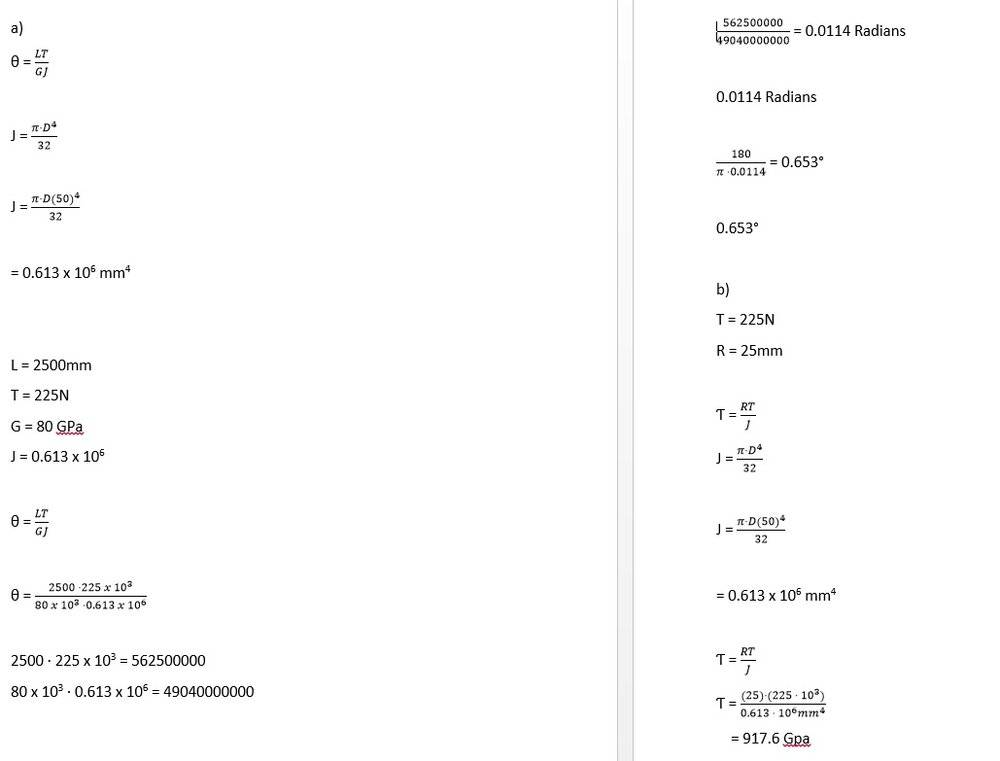Any help much appreciated.

It is not shown in the diagram, but the 225 N is a force, not a torque, and it acts tangent to the circumference of the shaft at its free end. What is the Torque caused by it? Then watch your conversion factors, there's a lot of zeros when using SI units.

•Lnewqban
So this whole calculation is wrong? Do i convert force to Torque? Thanks for replying to help

If you determine the correct torque and use it in your equations and check your math, and units, you will have it right!

•Ben_Walker1978 and Chestermiller
Ok.

So i have:

T = r x F

T = 25mm x 2.5N

T = 5,625Nmm

Is this correct calculations for torque?

So i can then place in my calculations and try complete the calculation.

Thank you.

Yes, That's T= (25)(225) = 5,625 N.mm

•Ben_Walker1978
Thank you.

I have re calculated.

Conversion factors is where i struggle.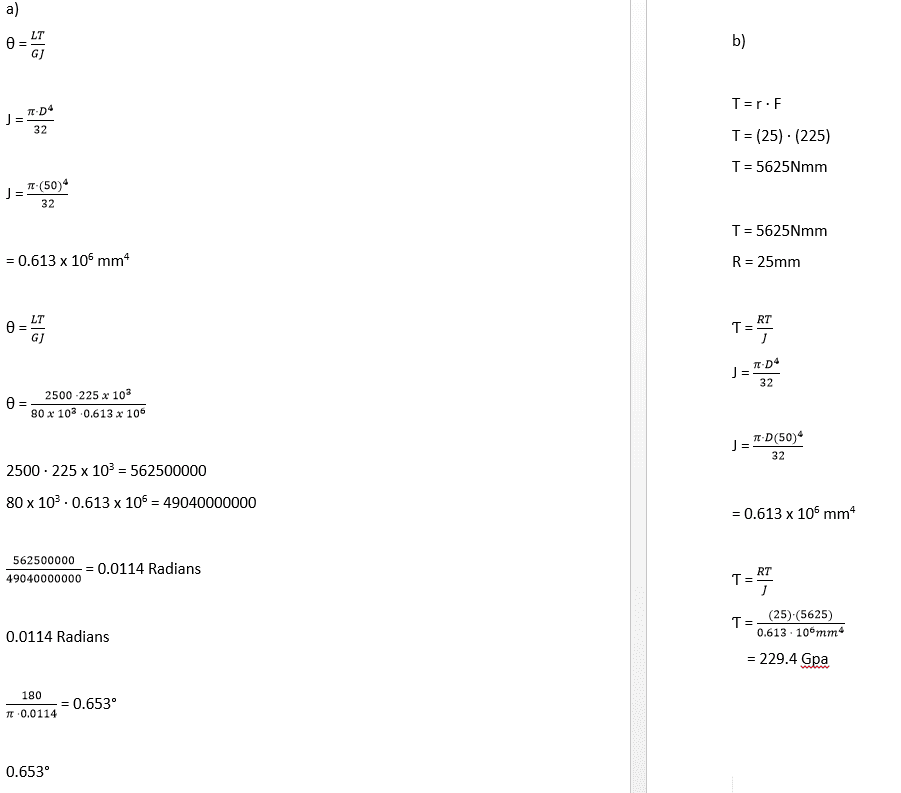Ben_Walker1978 said:
Conversion factors is where i struggle.
That’s for sure. Your answer for torsional shear stress is off by a factor of one million. Don’t feel bad, I’m from the US and I seldom use metric , so it confuses the living daylights out of me. Once I ordered 1/4 inch thick plate from China and they offered me 6mm instead. It took me awhile to convince myself that it was more or less the same. Except a lot cheaper than steel from the US mills.

And you didn’t correct your angle of twist!

I hope you get it right next time, because it takes me forever to do the math because of all those zeroes and mega and giga stuff.•Ben_Walker1978
I thought angle of twist was correct. Not sure where i have gone wrong there?

I was have another attempt and post.

I hope i get it right this time too!

Thank you for your help. Very much appreciated.

My attempt with new calculations.

I think this is now correct.

If not where am i going wrong?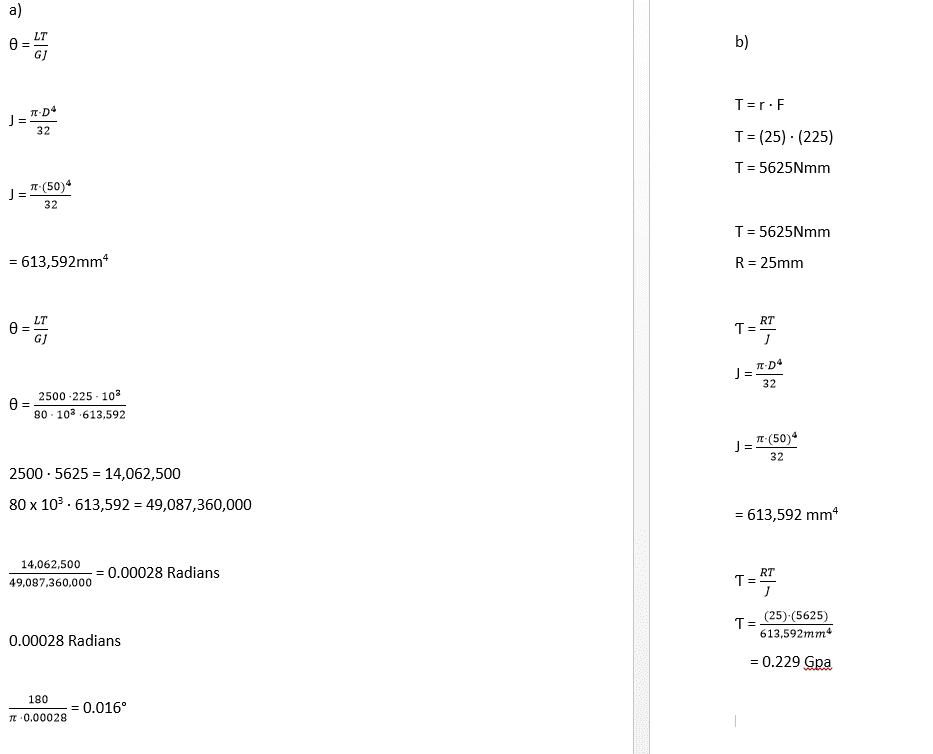•Lnewqban
Ben_Walker1978 said:
My attempt with new calculations.

I think this is now correct.

If not where am i going wrong?

The numerical values are correct this time, however, the maximum shear stress near the surface of the shaft should be 0.229 MPa (millions of Pascals) rather than GPa (billions of Pascals).

Ben_Walker1978 said:
Conversion factors is where i struggle.
My suggestion is that, before attempting any calculation, you convert all the given units to base and derived SI units.
Then, convert prefixes (like giga en GPa) to scientific notation.
By doing so, you very much simplify your calculations, since you only have integer powers of ten to deal with.

https://en.wikipedia.org/wiki/International_System_of_Units

https://en.wikipedia.org/wiki/Scientific_notation

Regarding the units, should should also keep track of the way they get combined in the several operations.
By doing so, you could catch errors at the end.

For example, you could have noted that your answer to a) in post #1 was not correct, unit-wise, as you entered N for torque rather than N-m and ended with an additional m that could not be cancelled.

Take a look at these examples of similar calculations (which use higher values of torque):
https://www.engineeringtoolbox.com/torsion-shafts-d_947.htmlLast edited:
•Ben_Walker1978
Lnewqban said:
The numerical values are correct this time, however, the maximum shear stress near the surface of the shaft should be 0.229 MPa (millions of Pascals) rather than GPa (billions of Pascals).

My suggestion is that, before attempting any calculation, you convert all the given units to base and derived SI units.
Then, convert prefixes (like giga en GPa) to scientific notation.
By doing so, you very much simplify your calculations, since you only have integer powers of ten to deal with.

https://en.wikipedia.org/wiki/International_System_of_Units

https://en.wikipedia.org/wiki/Scientific_notation

Regarding the units, should should also keep track of the way they get combined in the several operations.
By doing so, you could catch errors at the end.

For example, you could have noted that your answer to a) in post #1 was not correct, unit-wise, as you entered N for torque rather than N-m and ended with an additional m that could not be cancelled.

Take a look at these examples of similar calculations (which use higher values of torque):
https://www.engineeringtoolbox.com/torsion-shafts-d_947.htmlThank you for this.

I am going to attempt another question to try and get use to doing the calculations.

So i have changed to Mpa.

So this is now correct? So i can use to help solve another question.

Your help is very much appreciated.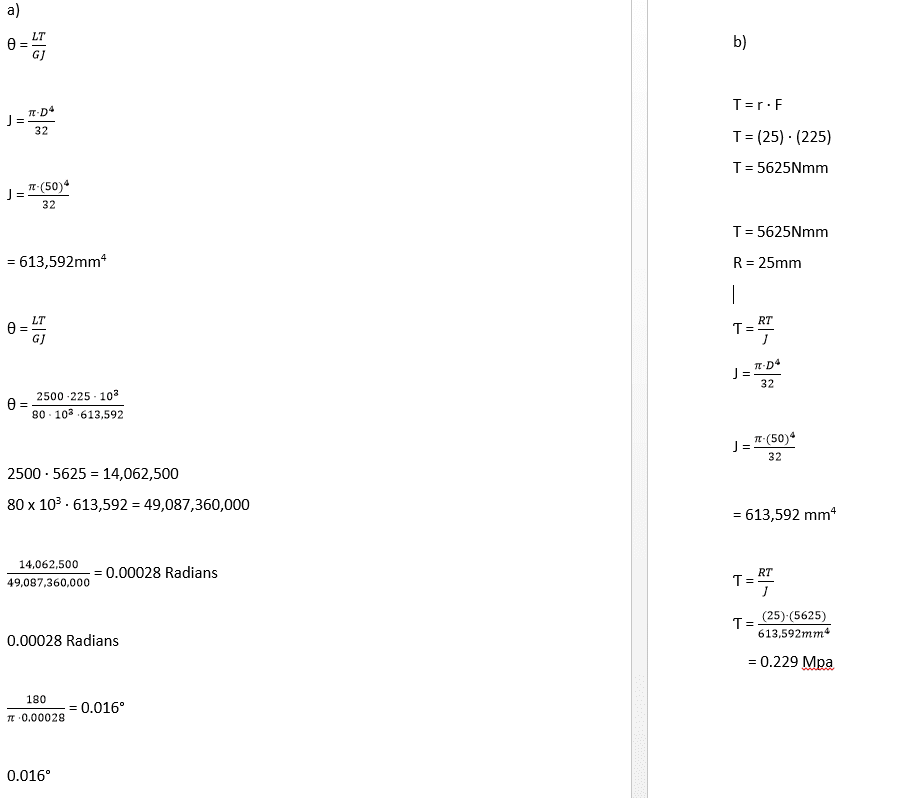Yes, I believe all is correct now.
You are very welcome, Ben.